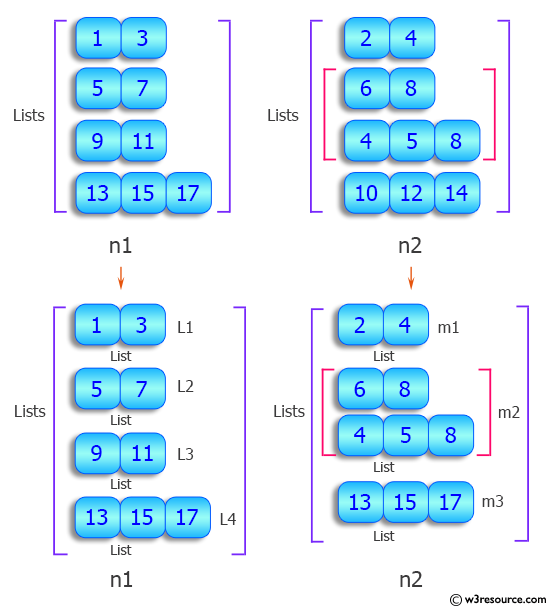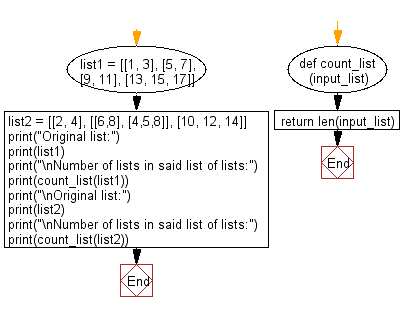﻿ Python: Count number of lists in a given list of lists - w3resource# Python: Count number of lists in a given list of lists

## Python List: Exercise - 90 with Solution

Write a Python program to count number of lists in a given list of lists.

Sample Solution:

Python Code:

``````def count_list(input_list):
return len(input_list)

list1 = [[1, 3], [5, 7], [9, 11], [13, 15, 17]]
list2 = [[2, 4], [[6,8], [4,5,8]], [10, 12, 14]]
print("Original list:")
print(list1)
print("\nNumber of lists in said list of lists:")
print(count_list(list1))
print("\nOriginal list:")
print(list2)
print("\nNumber of lists in said list of lists:")
print(count_list(list2))
```
```

Sample Output:

```Original list:
[[1, 3], [5, 7], [9, 11], [13, 15, 17]]

Number of lists in said list of lists:
4

Original list:
[[2, 4], [[6, 8], [4, 5, 8]], [10, 12, 14]]

Number of lists in said list of lists:
3
```

Pictorial Presentation:Flowchart:## Visualize Python code execution:

The following tool visualize what the computer is doing step-by-step as it executes the said program:

Python Code Editor:

Have another way to solve this solution? Contribute your code (and comments) through Disqus.

What is the difficulty level of this exercise?

Test your Python skills with w3resource's quiz

﻿

## Python: Tips of the Day

Floor Division:

When we speak of division we normally mean (/) float division operator, this will give a precise result in float format with decimals.

For a rounded integer result there is (//) floor division operator in Python. Floor division will only give integer results that are round numbers.

```print(1000 // 300)
print(1000 / 300)```

Output:

```3
3.3333333333333335```